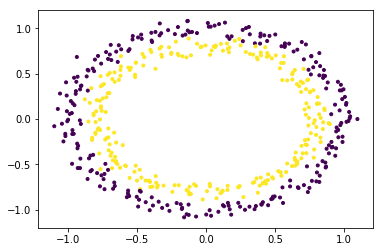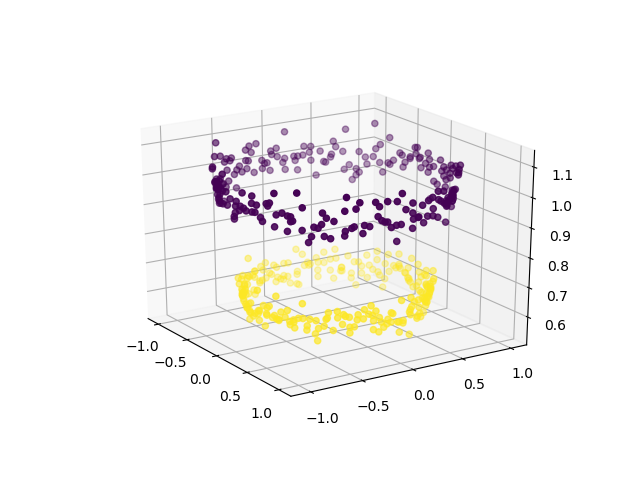top of page
Search

# SVM and the Maths behind it

Written by Rahul Rustagi

If you are one of those who have any experience in the field of Machine Learning or Data Science, you might have heard of many terms such as linear Regression, CNN, Classification etc. One such term which is quite famous and used around in the Data Science Community is SVM. In this article, we are going to dive deep into what SVM is and why anyone who is looking for a career in Data Science should know about it.

SVM stands for support vector machine, which I know doesn’t explain much but as you read this article you will gain some insight into SVM and how it works even if you are a beginner.

What is Support Vector Machine?

Support Vector Machine is a simple algorithm which is used mainly in classification problems where the target variable is a categorical one, but don’t we already have logistic regression for that or even decision trees, well here are some advantages why the SVM algorithm is preferred for many classification problems and sometimes even for regression too: -

• SVM has L2 Regularization feature. So, it has good generalization capabilities which prevent it from over-fitting.

• SVM can efficiently handle non-linear data using Kernel trick.

• Solves both Classification and Regression problems: SVM can be used to solve both classification and regression problems. SVM is used for classification problems while SVR (Support Vector Regression) is used for regression problems.

• A small modification in the dataset does not greatly affect the hyperplane and hence the SVM is relatively stable.

So many advantages do not mean it doesn’t have its fair share of disadvantages either, some of them are: -

• Complex and memory heavy calculations for the algorithmic functions

• Time consumed for large datasets is too high compared to its counterparts

• It is not a simple task to pick a suitable Kernel feature (to manage non-linear data). It Can be difficult and challenging. When you have a high-dimensional kernel, you may create so many support vectors that dramatically lower the training pace.

How does SVM work?

Enough with the advantages and disadvantages, let’s dive deep into how the SVM actually works and what makes it one of the most fascinating algorithms in the ml collective.

Here is how SVM actually works, it uses complex calculations to find the best suitable hyperplane to differentiate between the classes. A Hyperplane can be anything for a data with two features a hyperplane would be a line, for three a plane and as we go on the dimensions increase with the number of features in our dataset.There are many potential hyperplanes but the optimum one is where the margin value is maximum as that would create a proper distinction within classes using the support vectors as seen in the above diagram. Support vectors can be termed as the boundary values of a cluster or a class which dictates the negative and positive hyperparameters for a specific dataset.

Not only this SVM can also be used on non-linear data using kernel functions which helps the model interpret that data as linear. Basically, what these kernels do is add another dimension to the data which makes it easier to access and classify the values into different classes. Let’s take a look at how it works and what are the end resultsIn the above example, you can see although the classes are clearly separable to us because of the colour, there is no way a 2-d hyperplane could be drawn to separate these two classes without having colossal error percentage, so what can be done, well here is where the kernel part comes in. After adding another dimension, the dataset looks somewhat like this: -Voila! now we can easily draw a 3-d hyperplane to set up the marginal distance between the two classes. Such problems can be solved using different types of kernels that are usable in the SVM algorithm thus making easily mixable classes distinctive on the graph.

Let's get a little deeper in the concepts of SVM.

The math behind SVM

Now as we are familiar with the theory of SVM, let's see how it works and what parameters are required by the algorithm to find the best hyperplane.

SVM problem can be formulated as,

```w.x+b≥1 for y=+1 class
w.x+b≤-1 for y= -1 class```

the above two equation can be written as:

`y(w.x+b)+1≥0 for y=+1 and -1 class.`

We have two hyperplane H1 and H2 passing through the support vectors of +1 and -1 class respectively. so,

```w.x+b=-1 :H1
w.x+b=1 :H2```

And the distance between H1 hyperplane and origin is (-1-b)/|w| and distance between H2 hyperplane and origin is (1–b)/|w|. So, margin can be given as

```
M=(1-b)/|w|-(-1-b)/|w|
M=2/|w|```

Where M is nothing but twice the margin. So margin can be calculated as 1/|w|. As optimal hyperplane maximizes the margin then SVM objective is boiled down to the fact of maximizing the term 1/|w|.

Stay tuned for the implementation part of the same in programming languages such as python, R, etc.

About the person behind the keyboard: Rahul is pursuing B.tech and parallelly he is also a data science intern. He is passionate about Machine Learning and NLP and is on his way to be a great blogger. If you guys want to contact him, just click on his name.